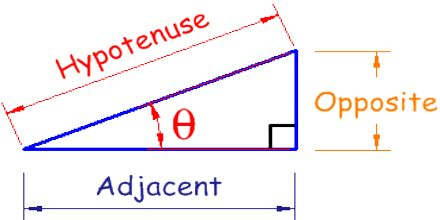Mathematic

# Trigonometry in 3DPrimary focus of this lecture is to present on Trigonometry in 3D. In a triangle, there are six basic elements: 3 sides and 3 angles. Not any three line segments may serve as the sides of a triangle. In modern trigonometry these relations are extended to arbitrary angles. This can be done, for example, by observing the projections of a rotating radius of a circle and a tangent at the end of the radius.